Last modification of this page:

### Errata Page for the Exercise Book

This is the errata web page for the book. If you find a mistake that is not already listed here, please report it to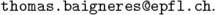The latest version of the errata can either be consulted on-line (see below) or downloaded as a [ps file] or as a [pdf file].

## 1  Prehistory of Cryptography

p. 8, Solution 1. In question 4, diagrams (a) and (c) do represent a surjective function.

## 2  Conventional Cryptography

p. 37, Solution 5. In question 1(a), one should read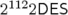and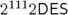for the worst-case and the average case repspectively.
p. 38, Solution 6. In the second question, the probability that a given plaintext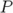is mapped on a given ciphertext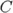through the uniformly distributed random permutation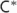should be expanded as follows: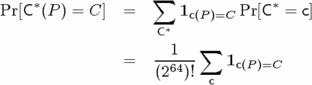p. 41, Solution 7. The errata for this exercise is long and only available in the postscript and pdf errata files (see on the top of this page).

## 3  Dedicated Conventional Primitives

p. 71, Solution 4. In the third question, one should read “Clearly, they all produce [...]” instead of “Clearly, the all produce [...]”.
p. 73, Solution 4. In the eighth question, one should read “with a probability e−λ” instead of “with a probability eλ”.

## 4  Conventional Security Analysis

p. 86, Exercise 7. The second of the three boolean functions is not used in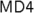It is actually part of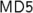which also uses a fourth function.
p. 109, Solution 8. On Figure 4.9, ω−1 is wrong: the inputs should be swapped before the xor.

Nothing yet.

## 6  Algorithmic Algebra

p. 147, Solution 5. In solution 3, one should read “the kernel is trivial, i.e., is equal to {1}”.

## 7  Algorithmic Number Theory

p. 170, Solution 5. The number of prime numbers smaller than some integer n is Ω(n/logn) and not Ω(logn/n) as written in the solution.

Nothing yet.

Nothing yet.

Nothing yet.

Nothing yet.

## 12  From Cryptography to Communication Security

p. 246, Solution 5. In the answer of the second question one should read P' = M' ∥ Q' instead of P' = M' ∥ Q.

## References

p. 250, Reference . There is a typo in the name of the first author. The correct name is P. Flajolet.

This document was translated from LATEX by HEVEA.